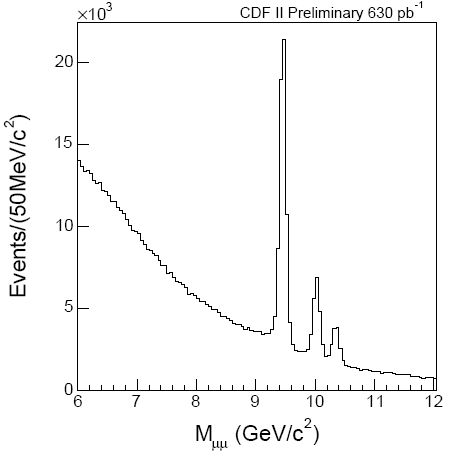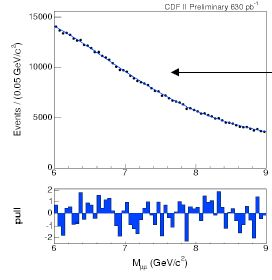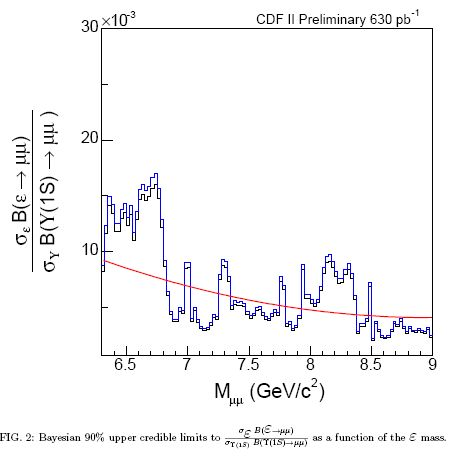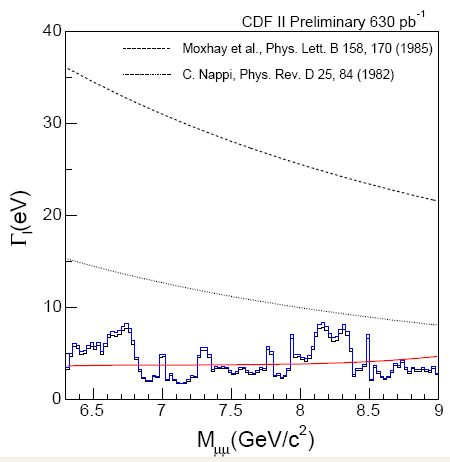## Do you remember the dimuon bump ? July 18, 2008

Posted by dorigo in news, physics, science.
Tags: , , ,

One year and a half ago I published here a series of posts where I discussed the story of the tentative observation of a new resonance in CDF. The signal appeared as a small bump in the mass distribution of pairs of muons: about 250+-60 events were fit on top of a smoothly falling background distribution, at a mass of 7.2 GeV. Here are the links to those posts:

The tentative signal was part of a larger package of anomalies that the CDF-Frascati group led by Paolo Giromini had put in evidence first in the sample of data which was the basis of the top pair cross section measurement, and later in another dataset containing semileptonic decays of b-hadrons.

I have told that story in detail in the series of five posts last year, but one detail was then still missing: was the search for the dimuon bump replicated in Run II ? After all, a signal whose significance was roughly of two standard deviations (don’t be fooled by arithmetics and check the posts linked above for a detailed explanation) found in a dataset of 90 inverse picobarns would become, if true, a towering peak with significance of six standard deviations in a dataset ten times larger.

Well, the analysis was indeed redone by the same group of physicists from Frascati, and the result is that the 7.2 GeV resonance was indeed a funny fluctuation.

Below you can see the relevant plots. The analysis is straightforward -just select events with two opposite-sign muon tracks, compute the invariant mass of the pair, and fit the distribution with a suitable function, inserting the degrees of freedom of a resonance fixing the mass in 25 MeV intervals. Extract the upper limit on the integral of the gaussian returned by the fit, and compute the resulting upper limit on the signal size as a function of mass. As easy as pie.Above you can see the mass distribution extracted from 630 inverse picobarns of data. No bump is apparent anywhere in the spectrum below 9 GeV, while above it the three beautiful signals of the Y(1S), Y(2S), and Y(3S) are unmistakable.On the left, you can see the mass region specifically considered by the analysis to search for narrow resonances. It is the same region where in Run I the 250-event bump had appeared. It is clear by visual inspection that any narrow resonance, if present in the data, is really small. The data is dominated by processes yielding muon pairs emitted in the decay of heavy quarks, with a significant contribution from fake muon backgrounds. The blue-bar “residual plot” shows the deviations from a smooth fit.The one above is the upper limit on the signal size as a function of its mass. The limit is computed as a fraction: the cross section for its production divided by the cross section for production of a Upsilon(1S), which is well-measured in its dimuon decay and has similar kinematical properties as the sought new state. Note that the vertical scale is in per mille units.And finally, the one above is the upper limit on the width of the resonance, compared to theory expectations (the hatched black curves). The fact that the limit is well below theoretical models implies that no such state can exist in the mass range examined.

The analysis solves a long-standing controversy. I must plaud to the scientific integrity of the group, who -after arguing for years about the possibility that something was indeed lurking in the Run I dimuon data- took the pains to demonstrate that this was indeed not the case as soon as they had enough Run II data to do so. Well done, folks!1. Kea - July 20, 2008